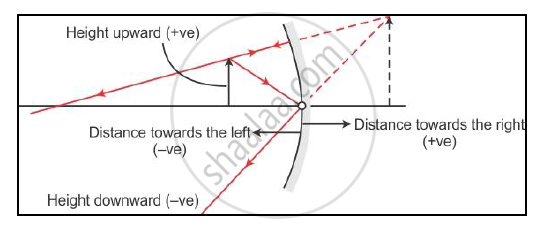# With the Help of an Appropriate Ray Diagram, State the Sign Conventions for Reflection by a Spherical Mirror. - Science and Technology 1

With the help of an appropriate ray diagram, state the sign conventions for reflection by a spherical mirror.

#### Solution

The sign convention for reflection by spherical mirrors is as follows:-1. The object is always placed to the left of the mirror.
2. All distances parallel to the principal axis are measured from the pole of the mirror.
3. All the distances measured to the right of the origin are taken as positive, while those measured to the left of the origin are taken as negative.
4. Distances measured perpendicular to and above the principal axis are taken as positive.
5. Distances measured perpendicular to and below the principal axis are taken as negative.
6. The focal length of a convex mirror is positive, while that of a concave mirror is negative.
Concept: Concave Mirror
Is there an error in this question or solution?